# MA 105 WEEK 7

Question 1

Evaluate the function at the indicated value of x.  Round your result to three decimal places.

Function: f(x) = 0.5x   Value: x = 1.7

 -0.308 1.7 0.308 0.5 -1.7

Question 2

Match the graph with its exponential function.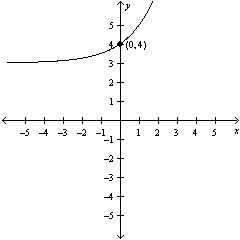y = 2-x - 3 y = -2x + 3 y = 2x + 3 y = 2x - 3 y = -2x - 3

Question 3

Select the graph of the function.

f(x) = 5x-1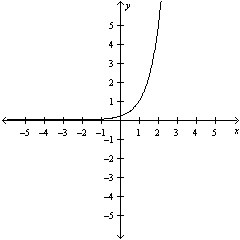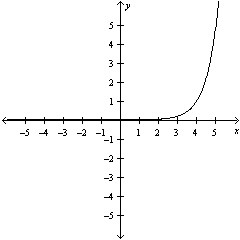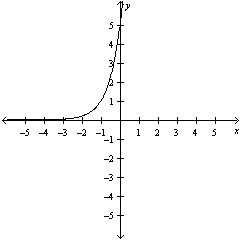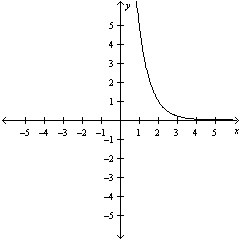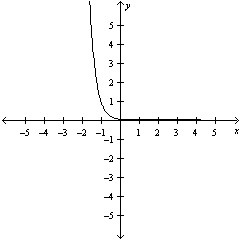Question 4

Evaluate the function at the indicated value of x.  Round your result to three decimal places.

Function: f(x) = 500e0.05x    Value: x=17

 1169.82 1369.82 1569.82 1269.82 1469.82

Question 5

Use the One-to-One property to solve the equation for x.

e3x+5 = e6

 x = -1/3 x2 = 6 x = -3 x = 1/3 x = 3

Question 6

Write the logarithmic equation in exponential form.

log8 64 = 2

 648 = 2 82 = 16 82 = 88 82 = 64 864 = 2

Question 7

Write the logarithmic equation in exponential form.

log7 343 = 3

 7343 = 2 73 = 77 73 = 343 73 = 14 3437 = 2

Question 8

Write the exponential equation in logarithmic form.

43 = 64

 log64 4 = 3 log4 64 = 3 log4 64 = -3 log4 3 = 64 log4 64 = 1/3

Question 9

Use the properties of logarithms to simplify the expression.

log20 209

 0 -1/9 1/9 -9 9

Question 10

Use the One-to-One property to solve the equation for x.

log2(x+4) = log2 20

 19 17 18 16 20

Question 11

Find the exact value of the logarithmic expression.

log6 36

 2 6 36 -2 none of these

Question 12

Use the properties of logarithms to expand the expression as a sum, difference, and/or constant multiple of logarithms.  (Assume all variables are positive.)

log3 9x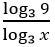log3 9 x log3 x log3 9 + log3 x log3 9 log3 none of these

Question 13

Condense the expression to a logarithm of a single quantity.

logx - 2logy + 3logz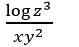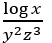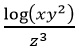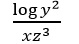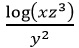Question 14

Evaluate the logarithm using the change-of-base formula.  Round your result to three decimal places.

log4 9

 1.585 5.585 3.585 4.585 2.585

Question 15

Determine whether the given x-value is a solution (or an approximate solution) of the equation.

42x-7 = 16

x = 5

 no yes

Question 16

Solve for x.

3x = 81

 7 3 4 -4 -3

Question 17

Solve the exponential equation algebraically.  Approximate the resulte to three decimal places.

e5x = ex2-14

 -7, -2 7, -2 5, -14 7, 2 -7, 2

Question 18

Solve the logarithmic equation algebraically.  Approximate the result to three decimal places.

log3(6x-8) = log3(5x + 10)

 18 20 17 19 -2

Question 19

Find the magnitude R of each earthquake of intensity I (let I0=1).

I = 19000

 3.28 5.28 4.28 2.38 6.28

Question 20

\$2500 is invested in an account at interest rate r, compounded continuously.  Find the time required for the amount to double.  (Approximate the result to two decimal places.)

r = 0.0570

 13.16 years 10.16 years 11.16 years 12.16 years
Field of study: